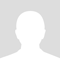# math algebra

The commutative property of addition says that you can add numbers in any order the commutative property of multiplaction is very similar it says that we can multiply numbers in any order with out changing the result addition
5a+4=
Multiplication 3x8x5b=

5a + 4 = 4 + 5a

And

3x8x5b = 3x5bx8 = 8x5bx3  ...

It's a super important property of algebra, Commutativity.

Commutativity allows mathematicians to prove all sorts of fancy theorems in mathematics. These proofs have implications on the physics and chemistry and biology that we use ubiquitously to improve the quality of life ... and educations.

Actually, Heisenberg's Uncertainty Principle from Quantum Mechanics can be seen to come from special cases where Commutativity doesn't work between fancy variables we use in Quantum Mechanics, called Operators. Operators represent measurements of physical quantities blah blah blah.\$20p/h

Charlton B.

charlton class room

if (isMyPost) { }